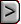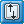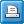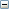﻿ DE4 - Direct Solver Package
Online Guide to MODFLOW

DE4 - Direct Solver PackageDE4 - Direct Solver PackageDE4 - Direct Solver PackageAbbreviation in Name file

DE4

Purpose

The Direct Solver package is used to solve the finite difference equations in each step of a MODFLOW stress period.

Documentation

 • Harbaugh, A.W., 1995, Direct solution package based on alternating diagonal ordering for the U.S. Geological Survey modular finite-difference ground-water flow model: U.S. Geological Survey Open-File Report 95-288, 46 p.
 • Harbaugh, A.W., Banta, E.R., Hill, M.C., and McDonald, M.G., 2000, MODFLOW-2000, the U.S. Geological Survey modular ground-water model -- User guide to modularization concepts and the Ground-Water Flow Process: U.S. Geological Survey Open-File Report 00-92, 121 p.
 • Harbaugh, A.W., 2005, MODFLOW-2005, the U.S. Geological Survey modular ground-water model -- the Ground-Water Flow Process: U.S. Geological Survey Techniques and Methods 6-A16. https://pubs.usgs.gov/tm/2005/tm6A16/
 • Mehl, S.W. and Hill, M.C., 2005, MODFLOW-2005, the U.S. Geological Survey modular ground-water model -- documentation of shared node local grid refinement (LGR) and the Boundary Flow and Head (BFH) Package: U.S. Geological Survey Techniques and Methods 6-A12, 68 p.

Related Packages

Solvers

Supported in

 • MODFLOW-2000
 • MODFLOW-2005
 • MODFLOW-LGR
 • MODFLOW-CFP
 • MODFLOW-NWT
 • MODFLOW-OWHM

Other Notes

If the Lake package is used, more iterations may be required.

Input Instructions

Input to the Direct Solver (DE4) Package is read from the file that is type "DE4" in the name file. All numeric variables are free format.

FOR EACH SIMULATION

Data Set 0

[#Text]

Item 0 is optional—“#” must be in column 1. Item 0 can be repeated multiple times.Text
 Text—is a character variable (199 characters) that starts in column 2. Any characters can be included in Text. The“#” character must be in column 1. Except for the name file, lines beginning with # are restricted to these first lines of the file. Text is printed when the file is read.

Data Set 1

ITMX MXUP MXLOW MXBWExplanation of variablesITMX
 ITMX—is the maximum number of iterations each time step. Specify ITMX = 1 if iteration is not desired. Ideally iteration would not be required for direct solution; however, it is necessary to iterate if the flow equation is nonlinear (see explanation for IFREQ = 3) or if computer precision limitations result in inaccurate calculations as indicated by a large water budget error. For a nonlinear flow equation, each iteration is equally time consuming because [A] is changed each iteration and Gaussian elimination is required after each change. This is called external iteration. For a linear equation, iteration is significantly faster because [A] is changed at most once per time step; thus, Gaussian elimination is required at most once per time step. This is called internal iteration.MXUP
 MXUP—is the maximum number of equations in the upper part of the equations to be solved. This value impacts the amount of memory used by the DE4 Package. If specified as 0, the program will calculate MXUP as half the number of cells in the model, which is an upper limit. The actual number of equations in the upper part will be less than half the number of cells whenever there are no-flow and constant-head cells because flow equations are not formulated for these cells. The DE4 Package prints the actual number of equations in the upper part when it runs. The printed value can be used for MXUP in future runs in order to minimize memory usage.MXLOW
 MXLOW—is the maximum number of equations in the lower part of equations to be solved. This value impacts the amount of memory used by the DE4 Package. If specified as 0, the program will calculate MXLOW as half the number of cells in the model, which is an upper limit. The actual number of equations in the lower part will be less than half the number of cells whenever there are no-flow and constant-head cells because flow equations are not formulated for these cells. The DE4 Package prints the actual number of equations in the lower part when it runs. The printed value can be used for MXLOW in future runs in order to minimize memory usage.MXBW
 MXBW—is the maximum band width plus 1 of the [AL] matrix. This value impacts the amount of memory used by the DE4 Package. If specified as 0, the program will calculate MXBW as the product of the two smallest grid dimensions plus 1, which is an upper limit. The DE4 Package prints the actual band width plus 1 when it runs. The printed value can be used for MXBW in future runs in order to minimize memory usage.

Data Set 2

IFREQ MUTD4 ACCL HCLOSE IPRD4Explanation of variablesIFREQ

IFREQ—is a flag indicating the frequency at which coefficients in [A] change. This affects the efficiency of solution; significant work can be avoided if it is known that [A] remains constant all or part of the time. If Local Grid Refinement is used, IFREQ should always be set equal to 3.

 • IFREQ = 1 indicates that the flow equations are linear and that coefficients of simulated head for all stress terms are constant for all stress periods. To meet the linearity requirement, all model layers must be confined (which is specified in the Block-Centered Flow Package by setting LAYCON equal to 0 for all layers or in the Layer-Property Flow Package by setting LAYTYP equal to 0 for all layers, or in the Hydrogeologic Unit Flow Package by setting LTHUF equal to 0 for all layers), and there must be no formulations that change based upon head (such as seepage from a river changing from head dependent flow to a constant flow when head drops below the bottom of the riverbed). Examples of coefficients of simulated head for stress terms are riverbed conductance, drain conductance, maximum evapotranspiration rate, evapotranspiration extinction depth, and general-head boundary conductance.
 • IFREQ = 2 indicates that the flow equations are linear, but coefficients of simulated head for some stress terms may change at the start of each stress period. (See IFREQ = 1 for information about linear equations.) Examples of coefficients of simulated head for stress terms are riverbed conductance, drain conductance, maximum evapotranspiration rate, evapotranspiration extinction depth, and general-head boundary conductance. For a simulation consisting of only one stress period, IFREQ = 2 has the same meaning as IFREQ = 1.
 • IFREQ = 3 indicates that a nonlinear flow equation is being solved, which means that some terms in [A] depend on simulated head. Examples of head-dependent terms in [A] are transmissivity for water-table layers, which is based on saturated thickness; flow terms for rivers, drains, and evapotranspiration if they convert between head dependent flow and constant flow; and the change in storage coefficient when a cell converts between confined and unconfined. When a nonlinear flow equation is being solved, external iteration (ITMX > 1) is normally required to accurately approximate the nonlinearities. Note that when nonlinearities caused by water-table calculations are part of a simulation, there are not necessarily any obvious signs in the output from a simulation that does not use external iteration to indicate that iteration is needed. In particular, the budget error may be acceptably small without iteration even though there is significant error in head because of nonlinearity. To understand this, consider the water-table correction for transmissivity. Each iteration a new transmissivity is calculated based on the previous head. Then the flow equations are solved, and a budget is computed using the new head with the same transmissivities. No budget discrepancy results because heads are correct for the transmissivity being used at this point; however, the new heads may mean that there should be a significant change in transmissivity. The new transmissivity will not be calculated unless there is another iteration. Therefore, when one or more layers is under water-table conditions, iteration should always be tried. The maximum change in head each iteration (printed by DE4 when IPRD4 = 1 and MUTD4 = 0) provides an indication of the impact of all nonlinearities.MUTD4

MUTD4—is a flag that indicates the quantity of information that is printed when convergence information is printed for a time step.

 • MUTD4 = 0 indicates that the number of iterations in the time step and the maximum head change each iteration are printed.
 • MUTD4 = 1 indicates that only the number of iterations in the time step is printed.
 • MUTD4 = 2 indicates no information is printed.ACCL
 ACCL—is a multiplier for the computed head change for each iteration. Normally this value is 1. A value greater than 1 may be useful for improving the rate of convergence when using external iteration to solve nonlinear problems (IFREQ = 3). ACCL should always be 1 for linear problems. When ITMX = 1, ACCL is changed to 1 regardless of the input value; however, a value must always be specified.HCLOSE
 HCLOSE—is the head change closure criterion. If iterating (ITMX > 1), iteration stops when the absolute value of head change at every node is less than or equal to HCLOSE. HCLOSE is not used if not iterating, but a value must always be specified.IPRD4
 IPRD4—is the time step interval for printing out convergence information when iterating (ITMX > 1). For example, if IPRD4 is 2, convergence information is printed every other time step. A value must always be specified even if not iterating.